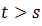# Four-Point Probe Method - Engineering Physics

Four-Point Probe Method

The Four-Point Probe method is used to measure the resistivity of a material. This method involves four equally spaced probes as shown in Figure which is in contact with the material of unknown resistance. The current is passing through the outer probes and voltage induces through the inner probes.

This method can be used either in bulk or thin specimen.

For Bulk -

Consider the material of thickness 't' when. Then the differential resistance due to the current through the outer probe is given byAs we know the current flow through two probes so resistance can be written as

So from eq. (1) and (2), we get

For Thin Sheet -
So in this case the deferential resistance is given by So from eq. (4) and (2), we get
It is independent of probe spacing.